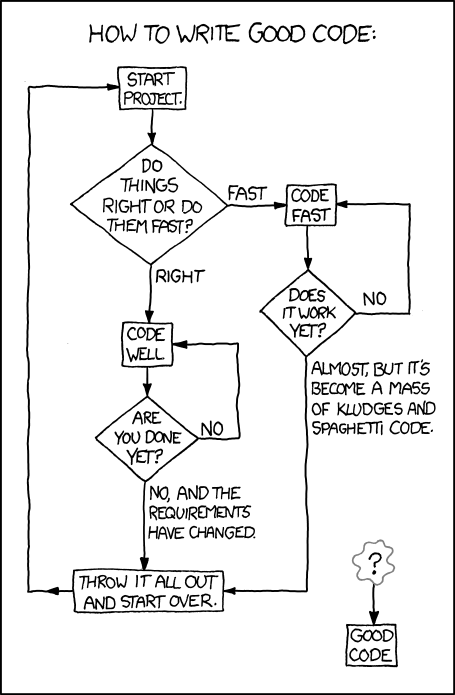← Back

January 7, 2011

# #844: Good Code((The comic is a flowchart. In order to explain this in text, follow the line numbers. Options follow on new lines without numbers.))

How to write good code.

((10.)) Start Project. ((Go to 20.))

((20.)) Do things right or do them fast?

Fast ((Go to 30.))

Right ((Go to 40.))

((30.)) Code fast. ((Go to 35.))

((35.)) Does it work yet?

No ((Go to 30.))

Almost, but it’s become a mass of kludges and spaghetti code. ((Go to 50.))

((40.)) Code well. ((Go to 45.))

((45.)) Are you done yet?

No. ((Go to 40.))

No, and the requirements have changed. ((Go to 50.))

((50.)) Throw it all out and start over. ((Go to 10.))

((60.)) ? ((Go to 70.))

((70.)) Good code.